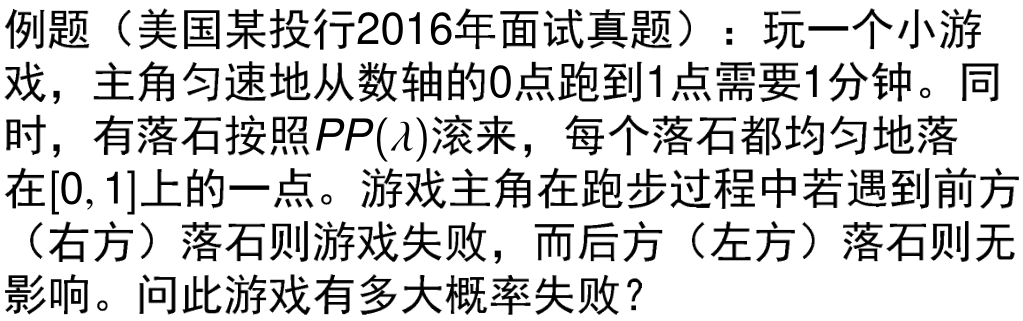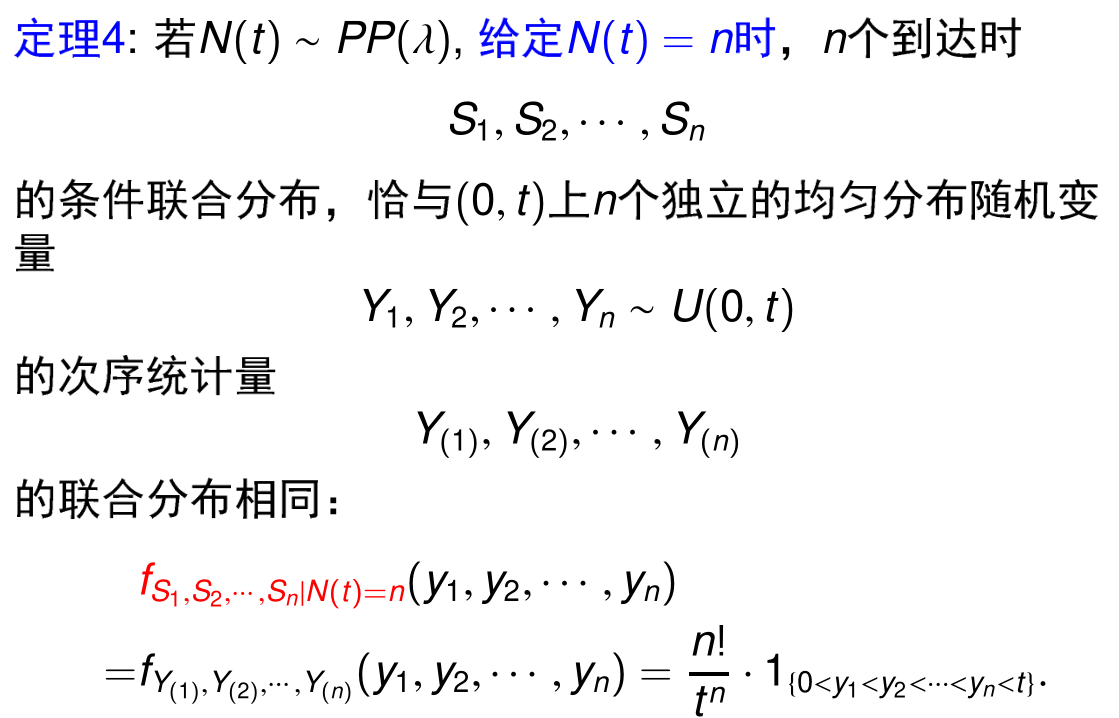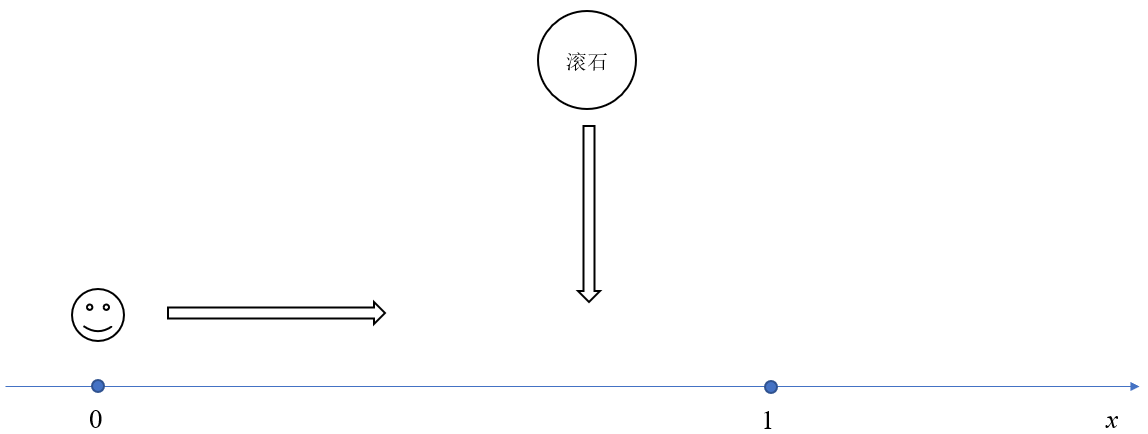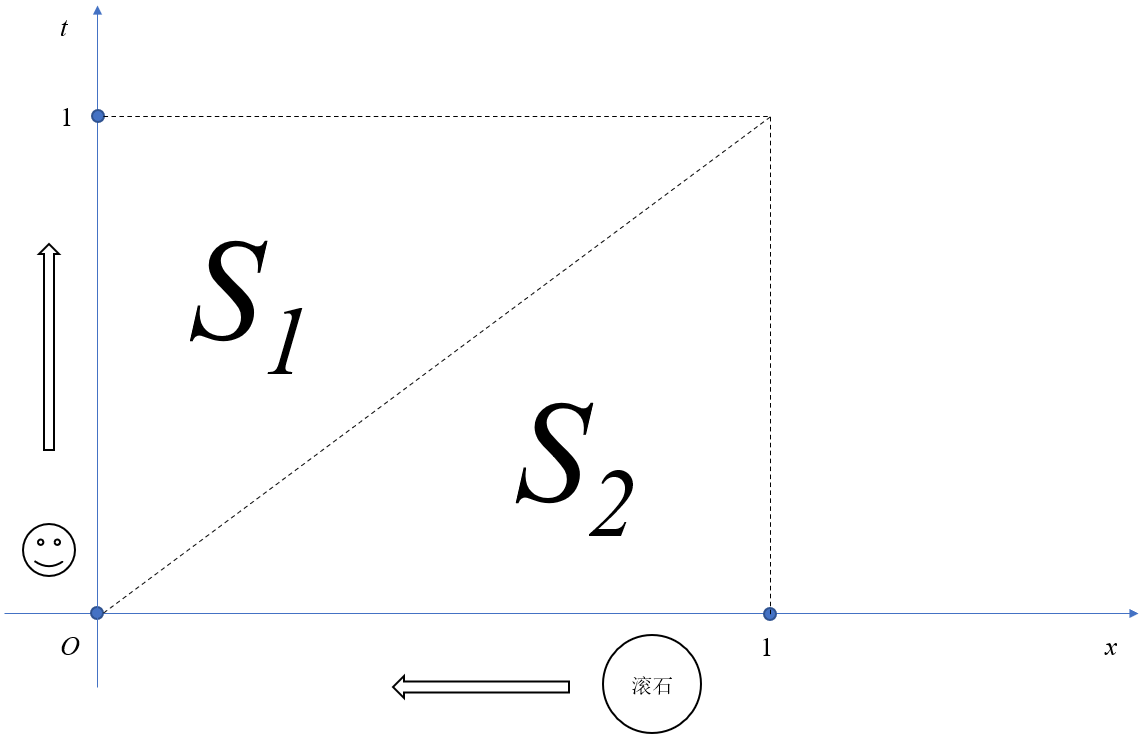# 序言其中 P P ( λ ) PP(\lambda) 指参数为 λ \lambda 的泊松过程。其中 N ( t ) N(t) 指在 [ 0 , t ] [0,t] 时间上泊松过程发生事件的次数， S i S_i 是第 i i 个事件发生的时刻；

# 解题思路事实上如果在上图中扩充一个时间维度，问题就变得豁然开朗：显然主角走完区间 [ 0 , 1 ] [0,1] 需要 1 1 单位的时间（ 1 1 分钟），滚石也只会落在 [ 0 , 1 ] [0,1] 的区间上，那么在什么情况下滚石会砸死主角？显然是当滚石掉落的坐标大于主角已经行走的时间，即滚石掉落的时间，在上图中即是 S 1 S_1 区域；

P ( s u r v i v e ) = ∑ n = 0 + ∞ ( 1 2 ) n ⋅ Pr ⁡ ( N ( 1 ) = n ) = ∑ n = 0 + ∞ ( 1 2 ) n ⋅ λ n n ! e − λ = e − λ 2 [ ∑ n = 1 + ∞ ( λ 2 ) 2 n ! e − λ 2 ] = e − λ 2 \begin{aligned} P(\rm survive)&=\sum_{n=0}^{+\infty}\left(\frac{1}{2}\right)^n\cdot\Pr\left(N(1)=n\right)\\ &=\sum_{n=0}^{+\infty}\left(\frac{1}{2}\right)^n\cdot\frac{\lambda^n}{n!}e^{-\lambda}\\ &=e^{-\frac{\lambda}{2}}\left[\sum_{n=1}^{+\infty}\frac{\left(\frac{\lambda}{2}\right)^2}{n!}e^{-\frac{\lambda}{2}}\right]\\ &=e^{-\frac{\lambda}{2}} \end{aligned}

# 后记

12-17689204-174395
07-061643
11-221026
02-078672
07-02682
11-111240
10-25840
08-131万+
02-181万+
04-13882
10-287063
02-181万+
11-053975
08-065万+